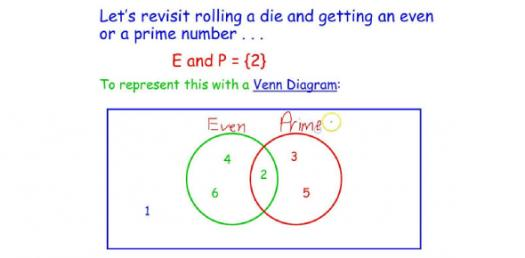# AP Statistics Practice Test! Trivia Quiz

5 Questions | Total Attempts: 605Settings.

Related Topics
• 1.
Moving times (in minutes) and weights (in pounds) were recorded for a random sample of 20 moving jobs requiring three-man crews, and the results of the regression analysis are shown below.  The equation for the least squares regression line is:
• A.

Predicted weight = 21.84 + 0.037(Time)

• B.

Predicted time = 21.84 + 0.037(Weight)

• C.

Predicted weight = 25.54 + 0.003(Time)

• D.

Predicted time = 25.54 + 0.003(Weight)

• E.

Predicted time = 0.037 + 21.84(Weight)

• 2.
The cause of death and the age of the deceased are recorded for 454 patients from a hospital.  Use these values to estimate the probability that a person at this hospital died as a result of an accident if it is known the person was between the ages of 45 and 54.
• A.

0.0264

• B.

0.0976

• C.

0.1322

• D.

0.2000

• E.

0.4878

• 3.
The median of a distribution is 150, and the interquartile range is 50.  Identify the statement(s) that must be true. (I). Approx. 50% of the data are between 125 and 175. (II). Approx. 50% of the data are less than or equal to 150. (III). Approx. 75% of the data are greater than 125.
• A.

I only

• B.

II only

• C.

I and III only

• D.

II and III only

• E.

I, II, and III

• 4.
For Hospital A, the average waiting time (time between walking in the door and seeing a doctor) in the emergency room is 135 minutes with a standard deviation of 45 minutes.  For Hospital B, the average waiting time in the emergency room is 90 minutes with a standard deviation of 22.5 minutes.  In which hospital are you more likely to wait less than 45 minutes?  Assume the distribution of waiting times is normal.
• A.

Hospital B, because the average wait-time is only 90 minutes, rather than 135 minutes in Hospital A.

• B.

Hospital A, because with twice the standard deviation of Hospital B, it has twice the spread.

• C.

Neither, because for both hospitals, the probability of waiting less than 45 minutes is 2.275%.

• D.

Neither, because for both hospitals, the probability of waiting less than 45 minutes is 97.725%.

• E.

It is impossible for any patient to wait less than 45 minutes in either hospital unless the patient is in critical condition.

• 5.
A regression equation is given as log(y-hat) = 0.214 - 1.28(x).  What is the (approximate) predicted value for y when x = 2?
• A.

- 2.346

• B.

- 0.171

• C.

0.005

• D.

0.167

• E.

Cannot be determined.